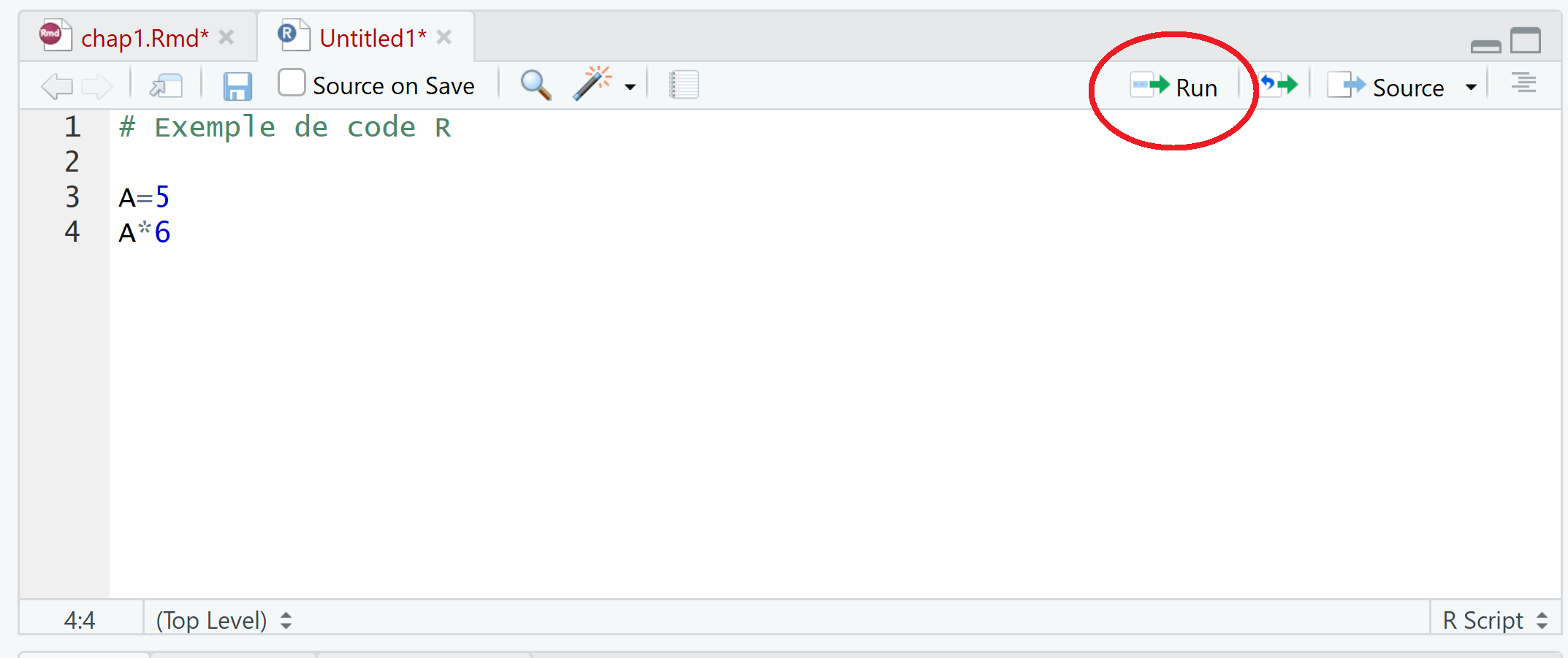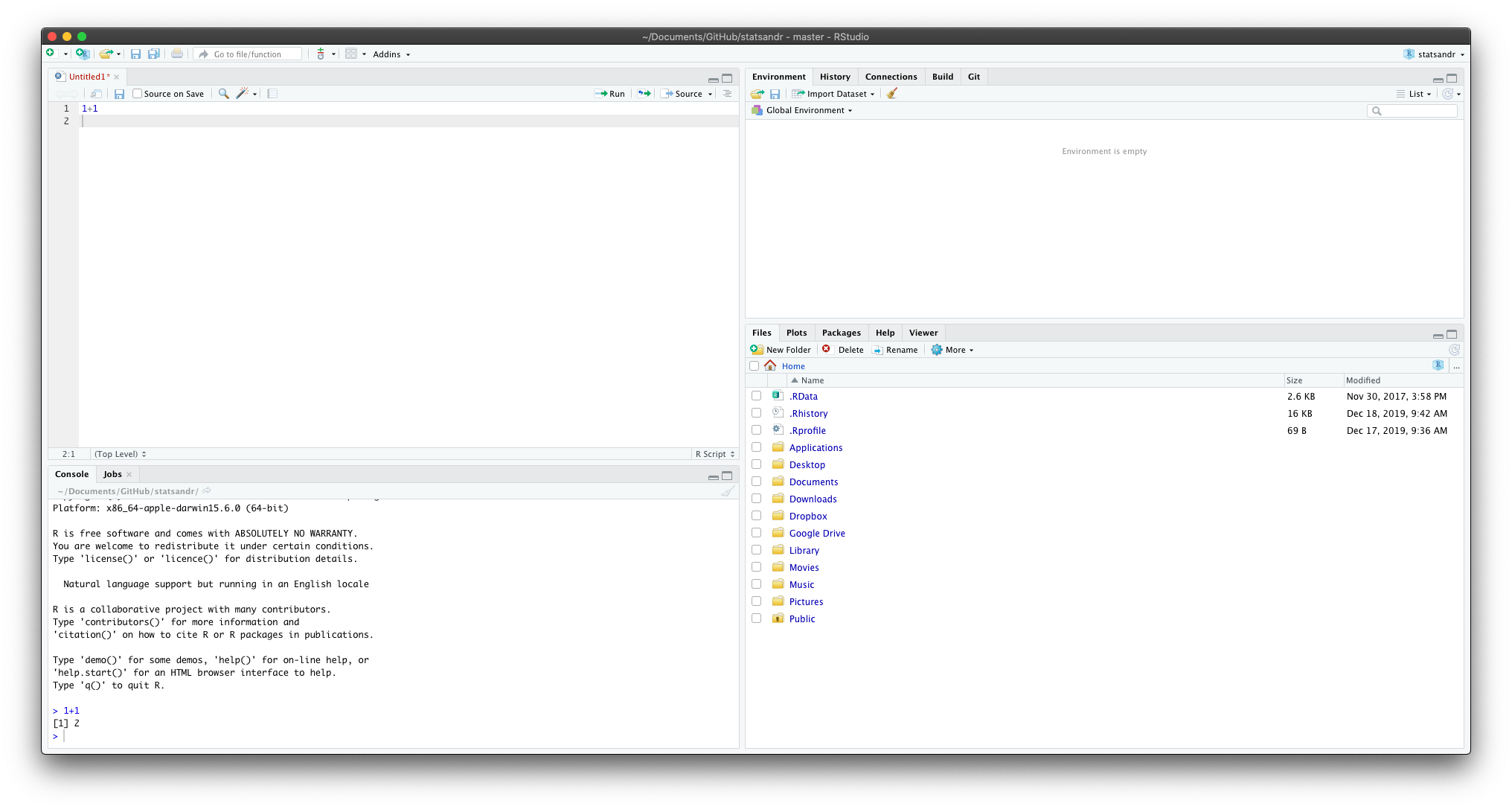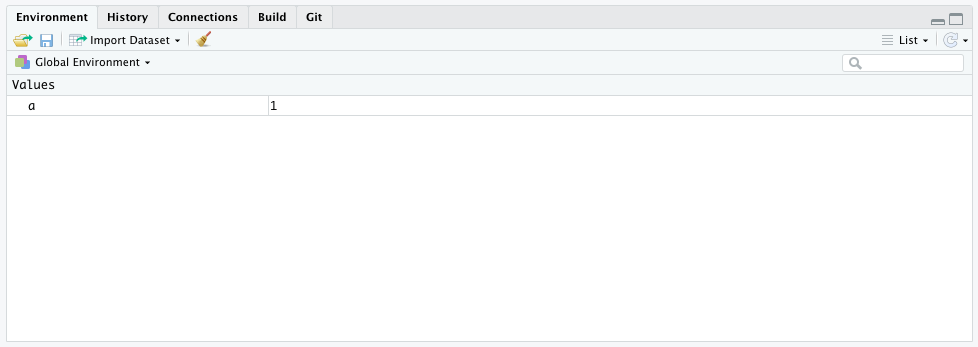Want to share your content on R-bloggers? click here if you have a blog, or here if you don't.

# What is R and RStudio?

## R

R is nothing more than a programming language. At the time of writing, this language is (one of) the leading program in statistics, although not the only programming language used by statisticians.

In order to use R, we need two things:

• a text editor in which to write our code
• a place to run this code

## RStudio

This is where RStudio comes handy. RStudio is an integrated development environment (IDE) for R. This software has the advantage of offering both a powerful text editor for writing your code and a place to run the code written in this editor (and I would add that it is more user-friendly). For these reasons, I highly recommend using RStudio instead of R. (Note that RStudio requires the prior installation of the R software provided by CRAN in order to be able to function properly. Just installing RStudio on your personal computer is not enough.)

# The main components of RStudio

Now that both programs are installed on your computer, let’s dive into the main components of RStudio.

By default, the RStudio window has three panes:

1. The console (red pane)
2. The environment (green pane)
3. Files, plots, help, etc. (blue pane)RStudio window

The console (red pane) is where you can execute your code (more information on the red and blue panes later). By default, the text editor does not open automatically. To open it, click on File > New File > R Script or click on the button representing a white sheet marked with a small green cross in the upper left corner, then on R Script:New R script in RStudio

A new pane (in orange below), also known as the text editor, opens in which you will be able to write your code. The code will be executed and the results displayed in the console (red pane).Write and execute your code in RStudio

Note that you can also write code in the console (red pane). However, I strongly recommend writing your code in the text editor (orange pane) because you can save the code written in the text editor (and thus execute it again later), while you cannot save the code written in the console.

To execute code written in the text editor (orange pane), you have two options:

• Type your code and then press the “Run” button (see below) or use the keyboard shortcut CTRL + Enter (cmd + Enter on Mac). Only the line of code where your cursor is located will then be executed
• Type your code and select in the text editor the part you want to execute and then press the “Run” button or use the keyboard shortcut CTRL + Enter (cmd + Enter on Mac). All the selected code will be executedRun code in RStudio

For example, try typing 1+1 in the text editor and execute it by clicking on “Run” (or CTRL/cmd + Enter). You should see the result 2 in the console, as the screenshot below:Example of code executed in RStudio

The text editor and the console are the panes you will use most ofen. The two other panes (the blue and green panes introduced earlier) will however still be very useful when using RStudio.

The environment (green pane) displays all values stored by RStudio. For example, if you type and execute the code a = 1, RStudio will store the value 1 for a, as the screenshot below:Environment pane in RStudio

This means that you can now perform any computations with a, such that if you execute a + 1, RStudio will render 2 in the console. In this pane you can also see a tab with a history of the code executed and a button to import a dataset (more on importing a dataset in RStudio).

The last pane (blue) is where you will find everything else such as your files, the plots, the packages, the help documentation, etc. I discuss about the Files tab in more details here so let’s discuss about the other tabs:

• Plot: where you will see the rendered plots. For instance, run plot(1:10) and you should see it in this tab
• Packages: where you see all your installed packages. Remind that RStudio is open source; everyone can write code and publish it as a package. You are then able to use this package (and all functions built inside this package) for free. Some packages are installed by default, others can be easily installed by running install.packages("name of the package") (do not forget "" around the name of the package!). Once the package is installed, you must load the package and only after it has been loaded you can use all the functions it contains. To load a package, run library(name of the package) (this time "" around the name of the package are not needed, but can still be used if you wish). Note that you will need to install packages only once, but load packages each time you open RStudio. Furthermore, note that an internet connection is required to install a package, while it is not to load a package
• Help: documentation about all functions written for R. To access the help of a function, run help("name of the function") or simply ?name of the function. For example, to see the help about the mean function, run ?mean

# Examples of code

Now that you have installed R and RStudio and you know its main components, below are some examples of basic code. More advanced code and analyses are presented in other articles about R.

## Calculator

Compute $$5*5$$

5 * 5

##  25

Compute $$\frac{1}{\sqrt{50\pi}}\, e^{-\frac{(10 – 11)^2}{50}}$$

1 / sqrt(50 * pi) * exp(-(10 - 11)^2 / 50)

##  0.07820854

As you can see, some values like $$\pi$$ are stored by default so you do not need to specify its value. Note that RStudio is case sensitive, but not space sensitive. This means that pi is different than Pi but 5*5 gives the same result than 5 * 5.

To add comments in your code, use # before the code:

# A comment
# Another comment
1 + 1

##  2

## Store and print values

Note that in order to store a value inside an object, using = or <- is equivalent. I however recommend using <- to follow the guidelines of R programming. You can name your objects (A and B in our case) as you like. However, it is recommended to use short and concise names (as you will most likely type them several times) and avoid special characters.

A <- 5
B <- 6

When storing values, RStudio does not display it on the console. To store a value AND print it in the console, use:

(A <- 5)

##  5

or:

A <- 5
A

##  5

## Vectors

It is also possible to store more than one value inside an object via the function c() (c stands for combine).

A <- c(1 / 2, -1, 0)
A

##   0.5 -1.0  0.0

## Matrices

Or create a matrix via matrix():

my_mat <- matrix(c(-1, 2, 0, 3), ncol = 2, nrow = 2)
my_mat

##      [,1] [,2]
## [1,]   -1    0
## [2,]    2    3

You can access the help of this function via ?matrix or help("matrix"). Note that inside a function, you can have multiple arguments separated by a comma. Inside matrix(), the first argument is the vector c(-1, 2, 0, 3), the second is ncol = 2 and the third is nrow = 2. For all functions in RStudio, you can specify an argument by its order inside the function or by the name of the argument. If you specify the name of the argument, the order does not matter anymore, so matrix(c(-1, 2, 0, 3), ncol = 2, nrow = 2) is equivalent to matrix(c(-1, 2, 0, 3), nrow = 2, ncol = 2):

my_mat2 <- matrix(c(-1, 2, 0, 3), nrow = 2, ncol = 2)
my_mat2

##      [,1] [,2]
## [1,]   -1    0
## [2,]    2    3

my_mat == my_mat2 # is my_mat equal to my_mat2?

##      [,1] [,2]
## [1,] TRUE TRUE
## [2,] TRUE TRUE

## Generate random values

To generate 10 values based on a normal distribution with mean $$\mu = 400$$ and standard deviation $$\sigma = 10$$:

my_vec <- rnorm(10, mean = 400, sd = 10)
# Display only the first 5 values:
head(my_vec, 5)

##  393.1760 398.1772 406.3960 391.8331 398.5543

# Display only the last 5 values:
tail(my_vec, 5)

##  393.1265 402.0290 400.2321 400.0694 406.6195

You will have different values than mines due to the fact that they are randomly generated. If you want to make sure to have always the same random values, use set.seed() (with any numeric inside the brackets). For instance, with the following code, you should have the exact same values, no matter where and when you run it:

set.seed(42)
rnorm(3, mean = 10, sd = 2)

##  12.741917  8.870604 10.726257

## Plot

plot(my_vec,
type = "l", # "l" stands for line
main = "Plot title",
ylab = "Y-axis label",
xlab = "X-axis label"
)This is only a very limited introduction to the possibilities of RStudio. If you want to learn more, I recommend that you read other articles related to R, starting with how to import an Excel file or how to manipulate a dataset.

Thanks for reading. I hope this article helped you to install R and RStudio. See other articles related to R here. As always, if you find a mistake/bug or if you have any questions do not hesitate to let me know in the comment section below, raise an issue on GitHub or contact me. Get updates every time a new article is published by subscribing to this blog.